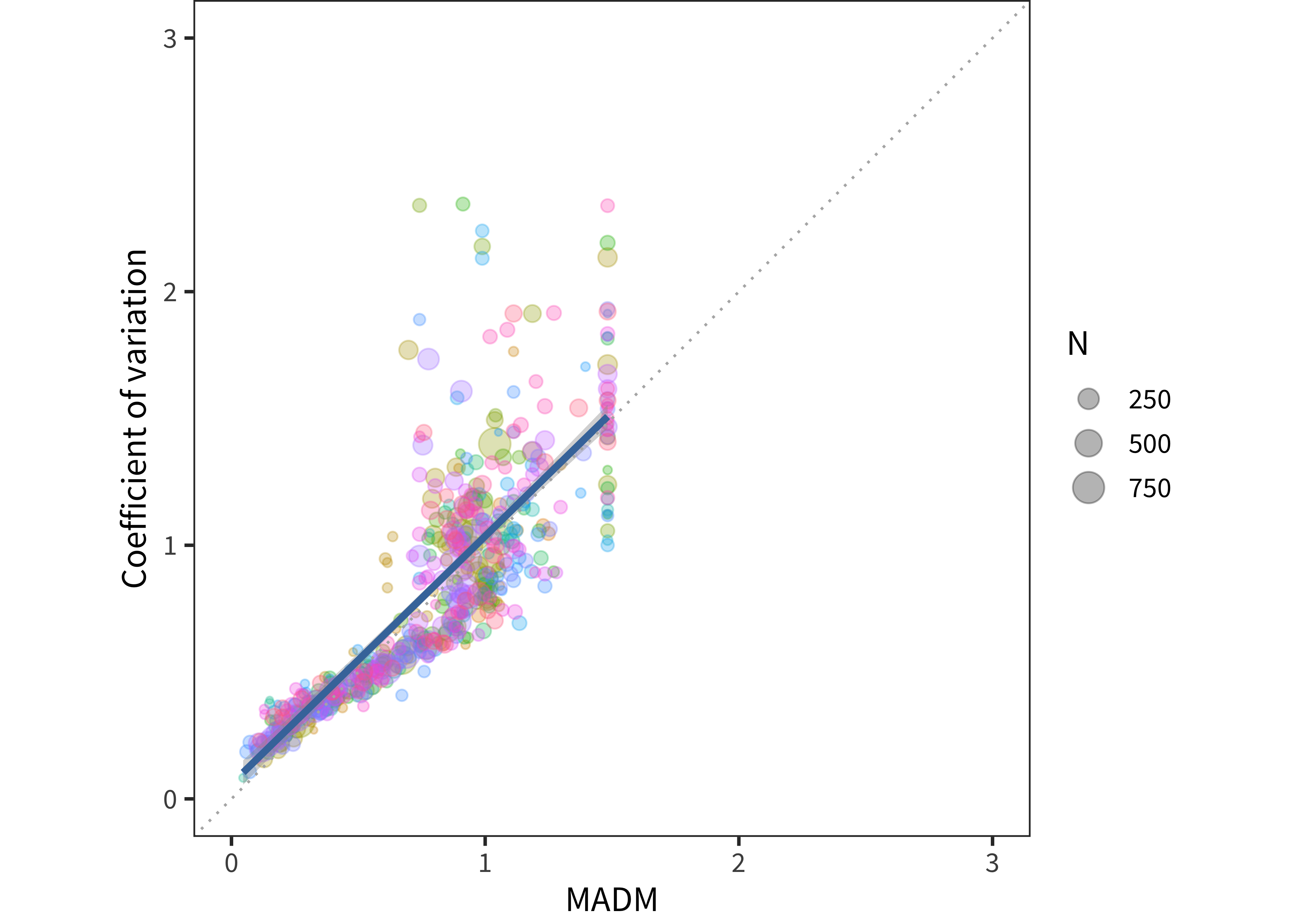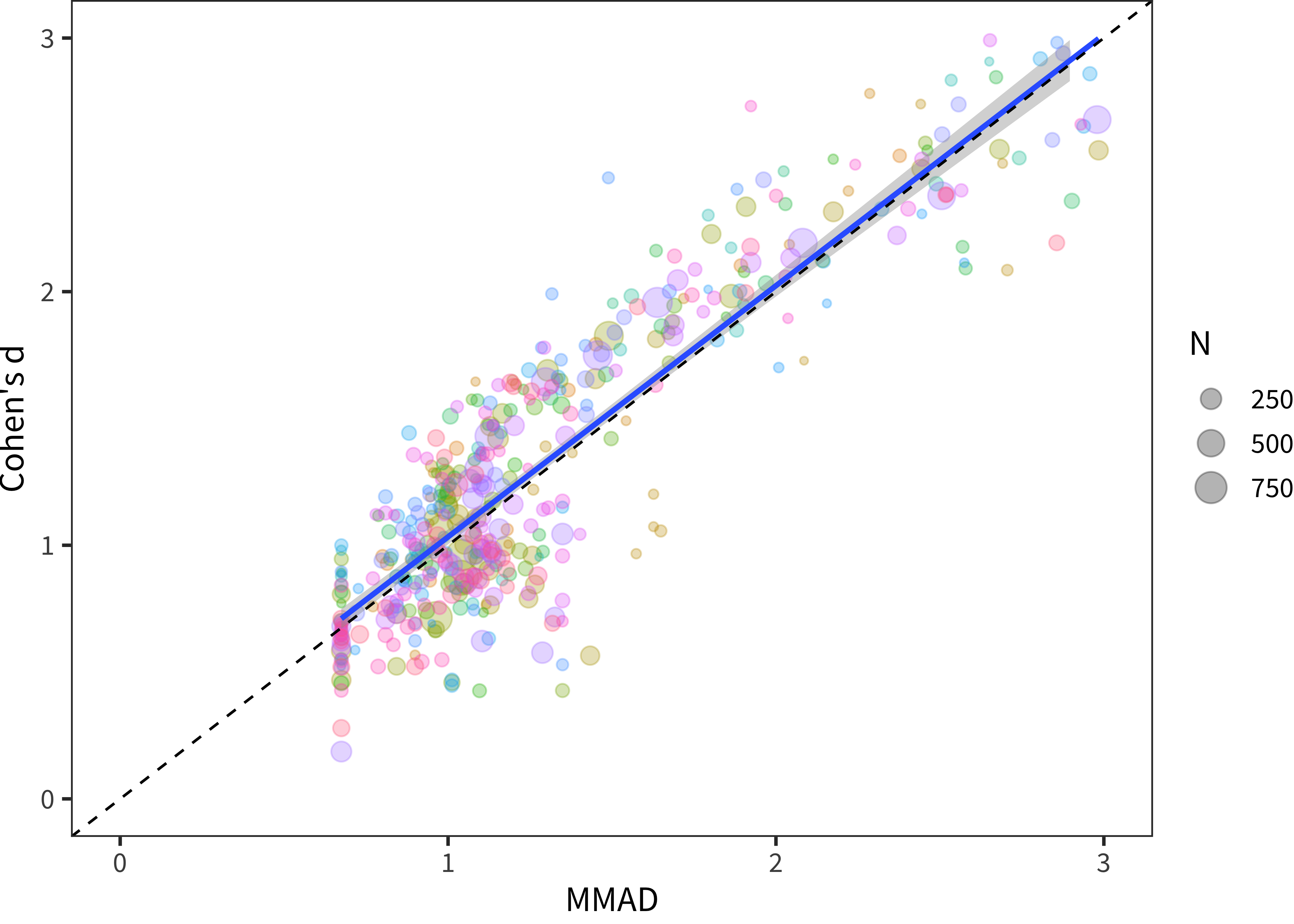# B Measures of Variability

In Chapter 5, we make use of non-parametric measures of variability, especially MADM (mean absolute deviation from the median) rather than the more standard coefficient of variation (CV). In this brief Appendix, we show that these measures are very highly correlated in the limit. We note however that the two measures (CV and MADM) produce quite different answers for individual data points, especially those that are at the floor or ceiling of a particular form.

Figure B.1 shows CV and MADM plotted together, with each point representing a single age group for a particular combination of form and language. The slope of the relationship between the two measures is strong ($$r$$ = 0.77) and its slope is close to 1, despite some variation. Overall, it appears that for the majority of the data, CV is slightly lower than MADM, but that it is dramatically higher for some individual datasets. We speculate that this relationship is due to floor/ceiling effects and small sample effects. This analysis suggests that MADM, the non-parametric estimate we use, is less subject to extreme fluctuations than CV.Figure B.1: Coefficient of variation and MADM, with each point showing a particular combination of language, form, and age and the line indicating a linear model fit.Figure B.2: Cohen’s d and MMAD, with each point showing a particular combination of language, form, and age and the line indicating a linear model fit.

Our second analysis, shown in Figure B.2, is identical except that it plots Cohen’s $$d$$ by MMAD. Each of these is the reciprocal of the related measure plotted above. (For example, $$d = \frac{\mu}{\sigma}$$ whereas $$CV = \frac{\sigma}{\mu}$$). Thus, the same relation holds.